#Method of Variation of ParametersThis method has no prior conditions to be satisfied. Therefore, it may sound more general than the previous method. We will see that this method depends on integration while the previous one is purely algebraic which, for some at least, is an advantage.

Consider the equation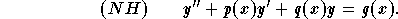In order to use the method of variation of parameters we need to know that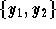is a set of fundamental solutions of the associated homogeneous equation y'' + p(x)y' + q(x)y = 0. We know that, in this case, the general solution of the associated homogeneous equation is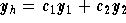. The idea behind the method of variation of parameters is to look for a particular solution such as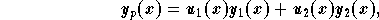where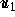and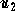are functions. From this, the method got its name.
The functionsandare solutions to the system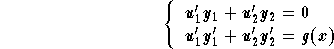,

which implies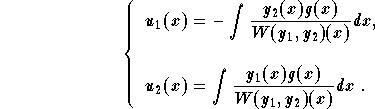,

where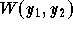is the wronskian of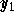and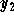. Therefore, we have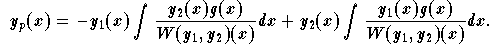Summary:Let us summarize the steps to follow in applying this method:

(1)
Finda set of fundamental solutions of the associated homogeneous equation

y'' + p(x)y' + q(x)y = 0.
;

(2)
Write down the form of the particular solution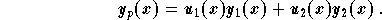;

(3)
Write down the system;

(4)
Solve it. That is, findand;
(5)
Plugandinto the equation giving the particular solution.

Example: Find the particular solution to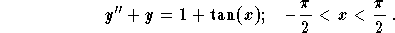Solution: Let us follow the steps:

(1)
A set of fundamental solutions of the equation y'' + y = 0 is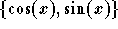;
(2)
The particular solution is given as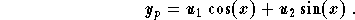(3)
We have the system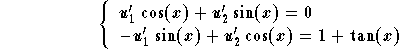;

(4)
We solve for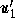and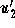, and get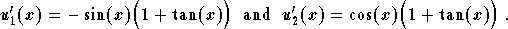Using techniques of integration, we get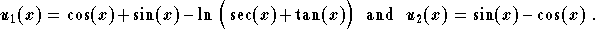;

(5)
The particular solution is: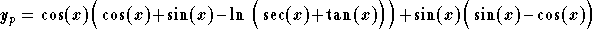,

or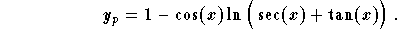Remark: Note that since the equation is linear, we may still split if necessary. For example, we may split the equation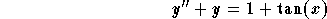,

into the two equations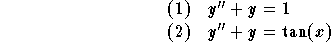then, find the particular solutionsfor (1) andfor (2), to generate a particular solution for the original equation by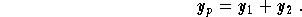There are no restrictions on the method to be used to findor. For example, we can use the method of undetermined coefficients to find, while for, we are only left with the variation of parameters.[Differential Equations] [First Order D.E.] [Second Order D.E.]
[Geometry] [Algebra] [Trigonometry ]
[Calculus] [Complex Variables] [Matrix Algebra]S.O.S MATHematics home page

Do you need more help? Please post your question on our S.O.S. Mathematics CyberBoard.Author: Mohamed Amine Khamsi